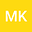Stochastic estimation of the distribution of soil water repellency on the soil surface in a humid-temperate forest
•## Abstract

Soil water repellency (SWR) increases surface runoff and preferential flows. Thus, quantitative evaluation of SWR distribution is necessary to understand water movements. Because the variability of SWR distribution makes it difficult to measure directly, we developed a method for estimating an SWR distribution index, defined as the areal fraction of surface soil showing SWR (SWRarea). The theoretical basis of the method is as follows: (1) SWRarea is equivalent to the probability that a position on the soil surface is drier than the critical water content (CWC); SWR is present (droplets absorbed in >10 s) when the soil surface is drier than the CWC and absent when it is wetter. (2) CWC and soil moisture content (θ) are normally distributed independent variables. (3) Thus, based on probability theory, the cumulative normal distribution of θ – CWC (f(x)) can be obtained from the distributions of CWC and θ, and f(0), the cumulative probability that θ – CWC < 0, gives the SWRarea. To investigate whether the method gives reasonable results, we repeatedly measured θ at 0–5 cm depth and determined the water repellency of the soil surface at multiple points in fixed plots with different soils and topography in a humid-temperate forest. We then calculated the CWC from the observed θ–SWR relationship at each point. We tested the normality of the CWC and θ distributions and the correlation between CWC and θ. Then, we determined f(x) from the CWC and θ distributions and estimated the SWRarea on each measurement day. Although CWC and θ were both normally distributed, in many cases they were correlated. Nevertheless, the CWC–θ dependency had little effect on the estimation error, and f(x) explained 69% of the SWRarea variability. Our findings show that a stochastic approach is useful for estimating SWRarea.
22 Dec 2021Submitted to Hydrological Processes
22 Dec 2021Submission Checks Completed
22 Dec 2021Assigned to Editor
22 Dec 2021Reviewer(s) Assigned
24 Jan 2022Review(s) Completed, Editorial Evaluation Pending
24 Jan 2022Editorial Decision: Revise Major